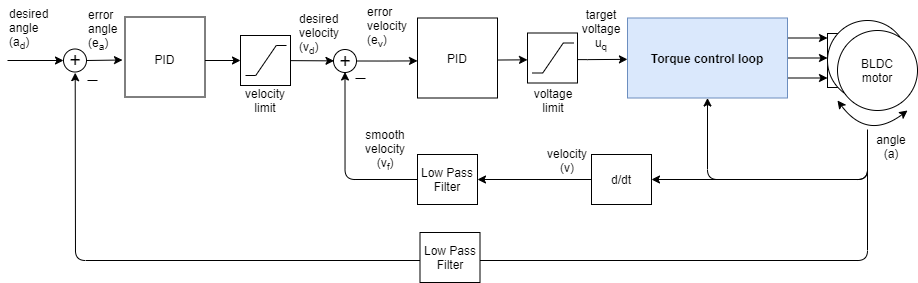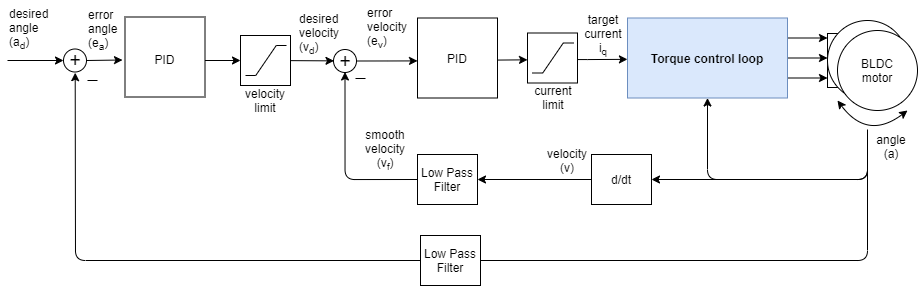# Position control loop

This control loop allows you to move your motor to the desired angle in real-time. This mode is enabled by:

// set angle/position motion control loop
motor.controller = MotionControlType::angle;


You can test this algorithm by running the examples in motion_control/position_motion_control/ folder.

## How it works

The angle/position control closes the control loop around the velocity control loop. And the velocity control closes the control loop around the torque control, regardless which one it is. If it is the voltage mode without phase resistance set, the velocity motion control will set the the torque command using the voltage Uq:And if it is any of the current torque control modes (FOC or DC current) or voltage mode with provided phase resistance, the angle motion control will be setting the target current iq to the torque controller:The angle control loop is therefore created by adding one more control loop in cascade on the velocity control loop like showed on the figure above. The loop is closed by using additional PID controller and an optional low pass filter. The controller reads the angle a from the motor (filters is optionally) and determines which velocity vd the motor should move to reach the desired angle ad set by the user. And then the velocity controller reads the current filtered velocity from the motor vf and sets the torque target (uq voltage or iq current) to the torque control loop, needed to reach the velocity vd, set by the angle loop.

## Controller parameters

To tune this control loop you can set the parameters to first velocity PID controller, low pass filter and the limits,

// velocity PID controller parameters
// default P=0.5 I = 10 D =0
motor.PID_velocity.P = 0.2;
motor.PID_velocity.I = 20;
motor.PID_velocity.D = 0.001;
// jerk control using voltage voltage ramp
// default value is 300 volts per sec  ~ 0.3V per millisecond
motor.PID_velocity.output_ramp = 1000;

// velocity low pass filtering
// default 5ms - try different values to see what is the best.
// the lower the less filtered
motor.LPF_velocity.Tf = 0.01;

// setting the limits
// either voltage
motor.voltage_limit = 10; // Volts - default driver.voltage_limit
// of current
motor.current_limit = 2; // Amps - default 0.2Amps


And then the angle PID controller, low pass filter and the limits:

// angle PID controller
// default P=20
motor.P_angle.P = 20;
motor.P_angle.I = 0;  // usually only P controller is enough
motor.P_angle.D = 0;  // usually only P controller is enough
// acceleration control using output ramp
// this variable is in rad/s^2 and sets the limit of acceleration
motor.P_angle.output_ramp = 10000; // default 1e6 rad/s^2

// angle low pass filtering
// default 0 - disabled
// use only for very noisy position sensors - try to avoid and keep the values very small
motor.LPF_angle.Tf = 0; // default 0

// setting the limits
//  maximal velocity of the position control
motor.velocity_limit = 4; // rad/s - default 20


It is important to parameter both velocity PID and angle PID controller to have the optimal performance. The velocity PID controller is parametrized by updating the motor.PID_velocity structure as explained in velocity control loop.

• Rough rule should be to lower the proportional gain P in order to achieve less vibrations.
• You probably wont have to touch the I or D value.

The angle PID controller can be updated by changing the motor.P_angle structure.

• In most applications just a simple P controller will be enough (I=D=0)
• Proportional gain P will make it more responsive, but too high value will make it unstable and cause vibrations.
• output_ramp value is the equivalent of the acceleration limit - default value is close to infinity, lower it if needed.

For the angle control you will be able to see the influence of the velocity LPF filter as well.

• The LPF_velocity.Tf value should not change much form the velocity control to the angle control. So once you have it tuned for the velocity loop you can leave it as is.
• The LPF_angle.Tf will in most cases remain equal to 0, which makes it disabled.

Additionally you can configure the velocity_limit value of the controller. This value prevents the controller to set too high velocities vd to the motor.

• If you make your velocity_limit very low your motor will be moving in between desired positions with exactly this velocity. If you keep it high, you will not notice that this variable even exists. 😃

Finally, each application is a bit different and the chances are you will have to tune the controller values a bit to reach desired behaviour.

## Position control example code

This is a very basic example of the position motion control program, based on voltage torque control with the complete configuration. When running this code the motor will move in between angles -1 RAD and 1 RAD each 1 sec.

#include <SimpleFOC.h>

// motor instance
BLDCMotor motor = BLDCMotor(11);
// driver instance
BLDCDriver3PWM driver = BLDCDriver3PWM(9, 10, 11, 8);

// encoder instance
Encoder encoder = Encoder(2, 3, 500);
// channel A and B callbacks
void doA(){encoder.handleA();}
void doB(){encoder.handleB();}

void setup() {

// initialize encoder sensor hardware
encoder.init();
encoder.enableInterrupts(doA, doB);
// link the motor to the sensor

// driver config
driver.init();

// set motion control loop to be used
motor.controller = MotionControlType::angle;

// controller configuration
// default parameters in defaults.h

// controller configuration based on the control type
// velocity PID controller parameters
// default P=0.5 I = 10 D =0
motor.PID_velocity.P = 0.2;
motor.PID_velocity.I = 20;
motor.PID_velocity.D = 0.001;
// jerk control using voltage voltage ramp
// default value is 300 volts per sec  ~ 0.3V per millisecond
motor.PID_velocity.output_ramp = 1000;

// velocity low pass filtering
// default 5ms - try different values to see what is the best.
// the lower the less filtered
motor.LPF_velocity.Tf = 0.01;

// angle P controller -  default P=20
motor.P_angle.P = 20;

//  maximal velocity of the position control
// default 20
motor.velocity_limit = 4;
// default voltage_power_supply
motor.voltage_limit = 10;

// use monitoring with serial
Serial.begin(115200);
// comment out if not needed
motor.useMonitoring(Serial);

// initialize motor
motor.init();
// align encoder and start FOC
motor.initFOC();

_delay(1000);
}

// angle set point variable
float target_angle = 1;
// timestamp for changing direction
long timestamp_us = _micros();

void loop() {

// each one second
if(_micros() - timestamp_us > 1e6) {
timestamp_us = _micros();
// inverse angle
target_angle = -target_angle;
}

// main FOC algorithm function
motor.loopFOC();

// Motion control function
motor.move(target_angle);
}


## Project examples

Here is one project example which uses position control and describes the full hardware + software setup needed.

Find more projects in the example projects section.